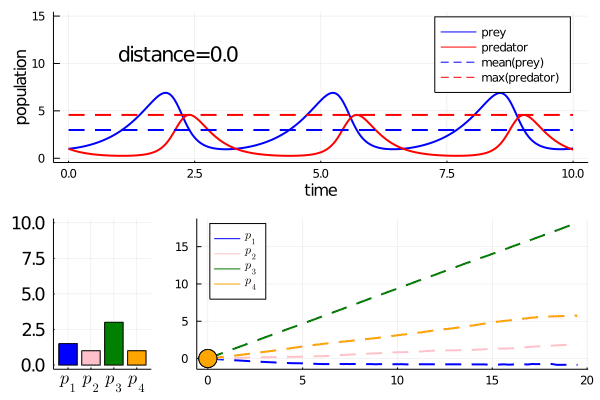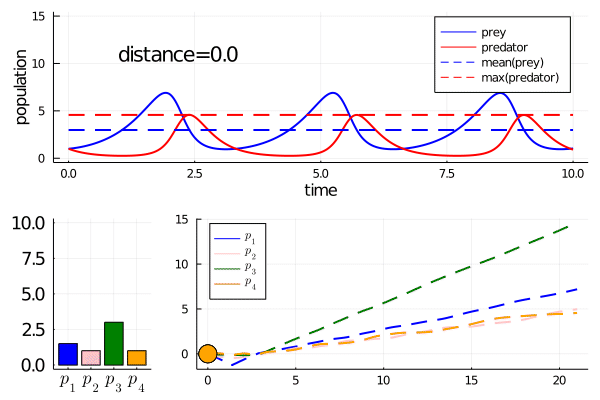# Examples(Credit: https://xkcd.com/1742/)

## Pulling notebooks from Github

Tutorial Pluto notebooks are in the MDCExamples repository.

1. At a terminal, in the directory of your choice, type the following commands:

git clone https://github.com/Dhruva2/MDCExamples.git
cd MDCExamples
julia

This clones the github repository with the examples, changes location to the cloned repository, and starts a julia session.

1. In your new julia session, open the Pluto environment:

using Pluto; Pluto.run()

1. Open and run the notebooks! Note that precompiling packages for the notebooks, the first time round, can take a few minutes. For information on how to run Pluto notebooks, see the docs

3b. Pluto notebooks run annotated .jl files. You can also run the .jl files themselves without using Pluto:

include("xxx.jl")

Suggested order to go through notebooks

1. Transforming_cost_functions.jl

2. mass_spring_intro.jl

3. NFKBExamples.jl

4. stg_neuron_prelim_collocation.ipynb

5. CircadianOscillator.ipynb

• No. 1 just shows you how to play around with cost functions

• No. 2 is an example on an extremely simple model. Running curves takes fractions of a second here, so it's a good playground for playing with curve settings.

• No. 3 is fairly detailed. It's useful if you want to see how scientific insight can be gained from looking at Minimally Disruptive Curves. Each curve on this notebook takes 2-10 mins to generate on my laptop (2017 macbook pro).

• Nos 4. and 5. show you how to use the (much quicker to evaluate) collocation cost. I've generated, but haven't really had time to properly analyse, the minimally disruptive curves.

## A copy-paste, minimal example

• This example is less didactic than the notebooks, but useful for somebody who just wants to get things working quickly.

• We do a cursory analysis of the Lotka-Volterra, predator-prey differential equation model. This is often used as a toy example for global sensitivity analysis tools, see e.g. the following web links: a, b, c. Because it's really simple.

• You can copy and paste the code at the bottom, and run it as a .jl script. It runs in seconds (after package/function pre-compilation). Just make sure you add all the package dependencies first:

] add OrdinaryDiffEq, ForwardDiff, Statistics, Plots, LinearAlgebra, LaTeXStrings
] add https://github.com/Dhruva2/MinimallyDisruptiveCurves.jl.git
• Note that one of the model features we analyse is non-differentiable. Mathematically. Computationally everything works fine, as we'll explain.

### Lotka Volterra model of predator-prey interactions

The dynamics for the system are:

$\frac{d 🐁}{dt} = p_1 🐁 - p_2🐁 🦉 \\ \frac{d 🦉}{dt} = -p_3🦉 + p_4 🐁 🦉$
• $p_1$ and $p_2$ are the birth and death rates of 🐁

• $p_3$ and $p_4$ are the birth and death rates of 🦉

... and here is a time-course of their populations when p = [1.5,1.0,3.0,1.0]. We call these values the nominal parameters.

Nominal system (i.e. simulated with nominal parameter values)• We will follow b in having two features of interest:

• mean population of 🐁 over time

• max population of 🦉 over time

• The model features of the nominal system are $[2.98, 4.57]$

• Our cost function on model behaviour is the mean square deviation from these nominal model features.

By the way In your ecology class, you may have heard of $r/K$ selection. This is a (criticised) theory of how there are two extremal strategies that individual species trade off between:

• r-Strategists (e.g. rabbits): high birth and death rate. low investment in individual organisms

• K-strategists (e.g. humans): low birth and death rate. high investment in individual organisms

We will use this terminology in our description of the MD curves.

• By running the code at the bottom of the page twice, setting whichdir = 1 and whichdir=2 respectively, we get two MD curves (see below).

### Minimally disruptive curve 1• As we move along the curve, predators move towards an $r$-strategy, and prey moves towards a $K$-strategy.

• We can also move in the opposite direction (prey to $r$ strategy, predator to $K$). To avoid figure clutter, run the pasted code yourself to see this!

### Minimally disruptive curve 2• This curve instead finds a way to modulate the frequency of predator prey interactions, while preserving model features

• Note how it abruptly changes direction when slowing frequency eventually prevents model features being maintained (as the period approaches the length of simulation). It goes from decreasing the frequency to increasing it.

### The code

• Gives static plots of the minimally disruptive curves. Code for the gifs was a bit more involved!

using OrdinaryDiffEq, ForwardDiff, MinimallyDisruptiveCurves, Statistics, Plots, LinearAlgebra, LaTeXStrings

#which md curve to plot
which_dir = 2

## define dynamics of differential equation
function f(du,u,p,t)
du = p*u - p*u*u #prey
du = -p*u + p*u*u #predator
end

u0 = [1.0;1.0] # initial populations
tspan = (0.0,10.0) #time span to simulate over
t = collect(range(0, stop=10., length=200)) # time points to measure
p = [1.5,1.0,3.0,1.0] # initial parameter values
nom_prob = ODEProblem(f,u0,tspan,p) # package as an ODE problem
nom_sol = solve(nom_prob, Tsit5()) # solve

## Model features of interest are mean prey population, and max predator population (over time)
function features(p)
prob = remake(nom_prob; p=p)
sol = solve(prob, Tsit5(); saveat = t)
return [mean(sol[1,:]), maximum(sol[2,:])]
end

nom_features = features(p)

## loss function, we can take as l2 difference of features vs nominal features
function loss(p)
prob = remake(nom_prob; p=p)
p_features = features(p)
loss = sum(abs2, p_features - nom_features)
return loss
end

loss(p)
end
return loss(p)
end

## package the loss and gradient into a DiffCost structure

"""
We evaluate the hessian once only, at p.
Why? to find locally insensitive directions of parameter perturbation
The small eigenvalues of the Hessian are one easy way of defining these directions
"""
hess0 = ForwardDiff.hessian(loss,p)
ev(i) = eigen(hess0).vectors[:,i]

## Now we set up a minimally disruptive curve, with nominal parameters p and initial direction ev(1)
init_dir = ev(which_dir); momentum = 1.; span = (-15.,15.)
curve_prob = MDCProblem(cost, p, init_dir, momentum, span)
@time mdc = evolve(curve_prob, Tsit5)

function sol_at_p(p)
prob = remake(nom_prob; p=p)
sol = solve(prob, Tsit5())
end

p1 = plot(mdc; pnames = [L"p_1" L"p_2" L"p_3" L"p_4"])

cost_vec = [mdc.cost(el) for el in eachcol(trajectory(mdc))]
p2 = plot(distances(mdc), log.(cost_vec), ylabel = "log(cost)", xlabel = "distance", title = "cost over MD curve");

mdc_plot = plot(p1,p2, layout=(2,1), size = (800,800))

nominal_trajectory = plot(sol_at_p(mdc(0.)[:states]), label = ["prey" "predator"])
perturbed_trajectory = plot(sol_at_p(mdc(-15.)[:states]), label = ["prey" "predator"])
traj_comparison = plot(nominal_trajectory, perturbed_trajectory, layout = (2,1), xlabel = "time", ylabel = "population")

### Lessons

• MinimallyDisruptiveCurves.jl showed how we could continuously vary the parameters of the Lotka-Volterra model, while preserving

1. mean prey population over time

2. max predator population over time

• One strategy (MDC1) was to alter the parameters to increase (decrease) birth and death rates of the predators while decreasing (increasing) those of the prey. Along the axis of $r$-strategies to $K$-strategies, this corresponds to one species moving in one direction, with the other going in reverse.

• Another strategy (MDC2) was to modulate the frequency of the oscillations. MDC2 showed us exactly how to change the parameters to continuously modulate this frequency.

By the way

The maximum predator population over time is a non differentiable function of the solution. How come this worked?

1. First note that ReLu units in neural networks are also technically nondifferentiable at a point, but are still used in differentiable machine learning models. Same reason!

2. OK the details? The maximum of a collection of elements (predator measurements over time), is differentiable except at crossing points, where two elements share a maximal value. Different values of predator(t) will never have exactly the same value, due to numerical error as well as equation dynamics. So it works out! Indeed, notice the timepoints at which maximal values are attained changes with the model parameters.